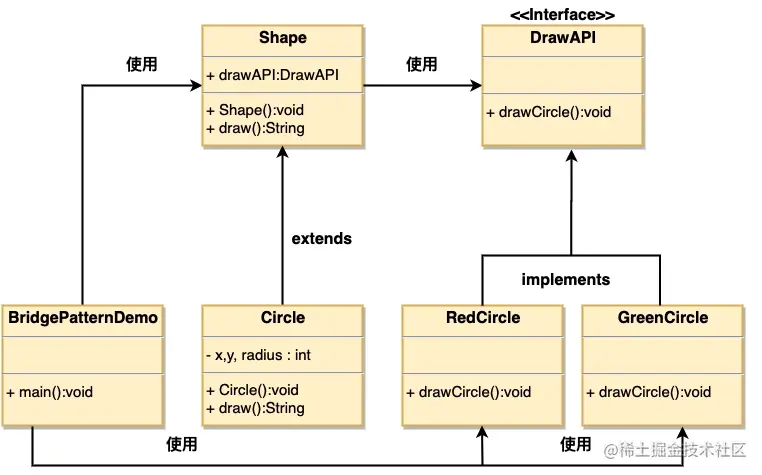## 介紹

## 實現

`DrawAPI` 接口定義了抽象方法，實現了 `DrawAPI` 接口的實體類 `RedCircle、GreenCircle``Shape` 是一個抽象類，將使用 `DrawAPI` 的對象。`BridgePatternDemo` 類使用 `Shape` 類來畫出不同顏色的圓。### 步驟1-創建接口類

``````// DrawAPI.java
public interface DrawAPI {
public void drawCircle(int radius, int x, int y);
}

### 步驟2-實現接口類

``````// RedCircle.java
public class RedCircle implements DrawAPI {
@Override
public void drawCircle(int radius, int x, int y) {
System.out.println("Drawing Circle[ color: red, radius: "
+ radius +", x: " +x+", "+ y +"]");
}
}

``````// GreenCircle.java
public class GreenCircle implements DrawAPI {
@Override
public void drawCircle(int radius, int x, int y) {
System.out.println("Drawing Circle[ color: green, radius: "
+ radius +", x: " +x+", "+ y +"]");
}
}

### 步驟3-創建形狀抽象類

``````// Shape.java
public abstract class Shape {
protected DrawAPI drawAPI;
protected Shape(DrawAPI drawAPI){
this.drawAPI = drawAPI;
}
public abstract void draw();
}

### 步驟4-創建形狀實現類

``````// Circle.java
public class Circle extends Shape {
public Circle(int x, int y, int radius, DrawAPI drawAPI) {
super(drawAPI);
this.x = x;
this.y = y;
}
public void draw() {
}
}

### 步驟5-設置場景類

``````// BridgePatternDemo.java
public class BridgePatternDemo {
public static void main(String[] args) {
Shape redCircle = new Circle(100,100, 10, new RedCircle());
Shape greenCircle = new Circle(100,100, 10, new GreenCircle());
redCircle.draw();
greenCircle.draw();
}
}

### 步驟6-輸出結果

``````Drawing Circle[ color: red, radius: 10, x: 100, 100]
Drawing Circle[ color: green, radius: 10, x: 100, 100]